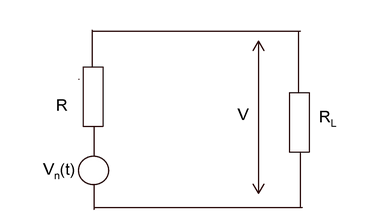# Noise voltage and absolute temperature

Q14. Noise voltage Vn and absolute temperature T are related as

Options:

a) Vn =1/ √(4RKTB)
b) Vn = √(4RK)/ (TB)
c) Vn = √(4RKTB)
d) Vn = √(4KTB)/R

Correct Answer: c) Vn = √(4RKTB)

Explanation :

In the following figure the maximum Noise power is given by Pn = V2/ RRL = R, for maximum power transfer from Vn to load resistor RL
In the above figure, according to voltage divider method,
V= Vn/2
Pn = V2/ R
= (Vn/2)2/ R
=Vn2/ 4R
Vn2= 4R Pn
Vn= √(4R Pn)
Noise power Pn= KTB
Where
K = 1.381×10-23 J/K, joules per Kelvin, the Boltzmann constant
B is the bandwidth at which the power Pn is delivered.
T noise temperature
Therefore, Vn= √(4R KTB)